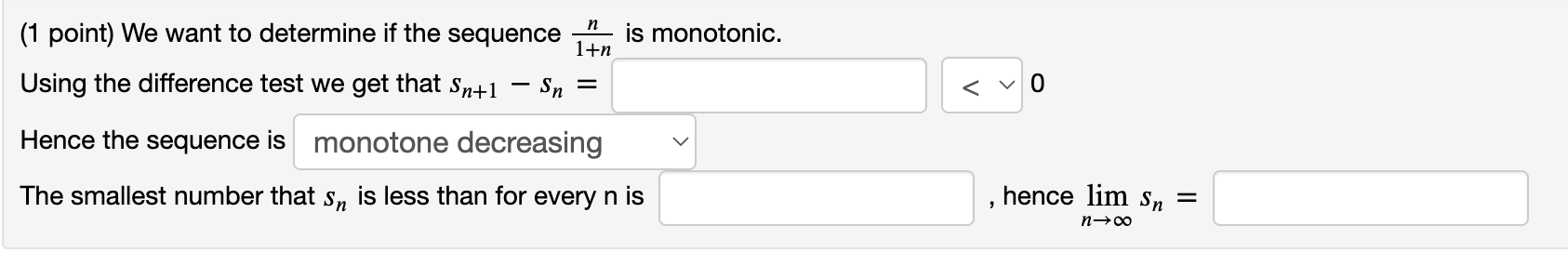Home / Expert Answers / Calculus / 1-point-we-want-to-determine-if-the-sequence-1-nn-is-monotonic-using-the-difference-test-we-pa649

# (Solved): (1 point) We want to determine if the sequence 1+nn is monotonic. Using the difference test we ...(1 point) We want to determine if the sequence is monotonic. Using the difference test we get that 0 Hence the sequence is The smallest number that is less than for every is , hence

We have an Answer from Expert